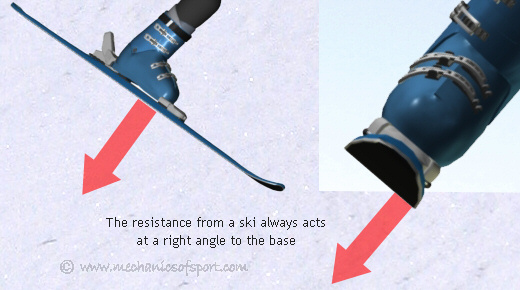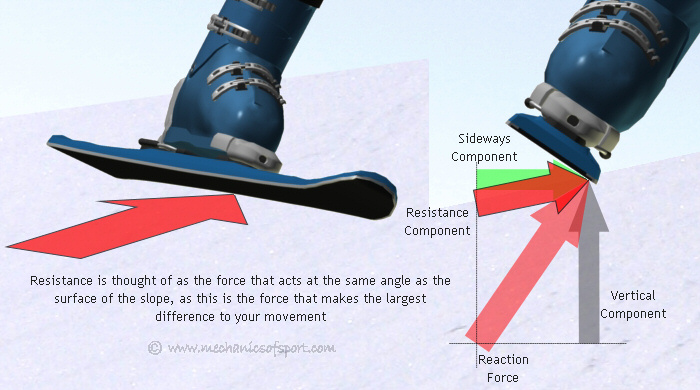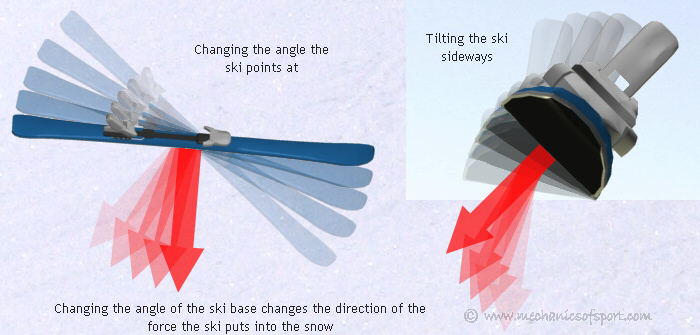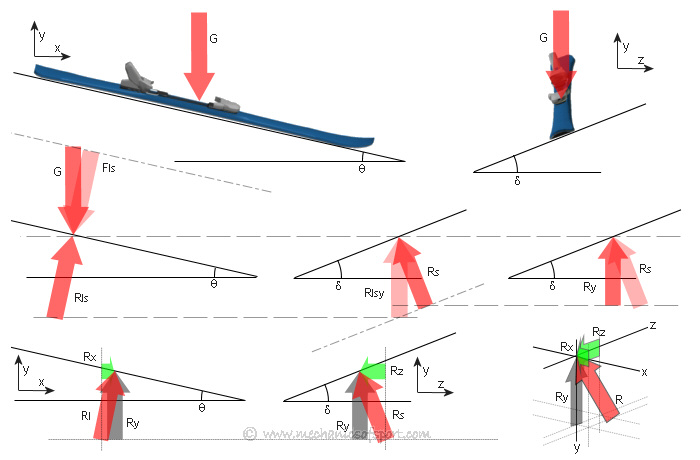SKIING

# Creating Resistance

By using edge pressure and pointing our skis in different directions, we can create different amounts of resistance in many different directions. It's this resistance that we use for all our control in skiing, so is useful to have an understanding of.

The first very important thing to know about creating resistance is that, as with all forces when we are not considering friction, the force a ski puts into the snow acts at a right angle to it's surface (its base). As shown by the diagram below.The reaction force also acts at a right angle to the base, but in the opposite direction. It is the reaction force that provides the ski's resistance, although we generally think of resistance as being the component of the reaction force that is acting along the surface of the slope, i.e. the component that is going to effect your velocity.## Directing Resistance

There are 2 things you can do with a ski which will effect the reaction force and its components. The first is to tilt a ski onto it's edge as we have gone through in edge pressure and edge effects, and the second is to change the angle that a ski is pointing in, along its length. Tilting a ski determines how large the sideways component of the reaction force can be, and changing the angle a ski points in, determines which direction the sideways component will act in.As a ski's resistance comes from the ski's reaction from the snow, in order to know what the ski's reaction will be, you need to know what forces are pushing the ski into the snow. There are 2 forces that can push the ski into the snow; gravity and a momentum induced force from changing your velocity. Gravity always acts straight downwards with a set force, and always needs to be accounted for as it is always present. The momentum induced force though, will only act if you are changing velocity. It's strength can vary, but it will always act in the same direction as your movement. It is the momentum induced force that can create G-forces as we turn. Put simply the more a ski is put so that it is sideways to the fall line, and our velocity, the more resistance it will create. Most of the time our velocity will be in a different direction to the fall line though, so there is almost always a compromise if you are trying to create as much resistance as possible. Normally we only create as much resistance as possible though if we are trying to stop, in any other situation we use resistance for control, which is covered further in the using resistance section.

## Calculating Resistance

To understand how the resistance you are creating acts, it is neccesary to know how forces effect each other and how they are calculated in different directions. To understand this perfectly is not important, as you are never going to try to actually calculate any forces, but to have a rough understanding will help you work out what effects different things will have. For the example we are ignoring friction, as with skiing it can generally be considered as negligible.

In skiing there are 3 different types of forces.

• Major forces - Major forces are the forces that create all other forces. They can act in any direction, as they are largely independent and act on a mass not a surface. They are created by an acceleration (either gravity, or a negative acceleration against movement).
• Minor forces - Minor forces are the forces that act on surfaces, and are made by the effects of major forces. They are created when a force is transfered through 2 surfaces pushing against each other, and always act at a right angle to a surface.
• Components - Components are not forces within their own right, but elements of major or minor forces that show how strong a force is in a certain direction. Any force can be shown as 3 component forces along the 3 axis.

Because all forces are created by major forces, major forces are the biggest forces, and can not be exceeded in any direction by minor forces or components. Whenever a major force is transmitted through a surface, the component of the force that is at a right angle to the surface, becomes a minor force. Any reaction from that force then has to be calculated from the minor force, as that is the actual physical force that would be present. Using newtons 3rd law every force has an equal and opposite reaction, so any force transmitted from a surface to another surface will have an even force transmitted back. This is the reaction force, and is the force of which its components can change our velocity.

So as an example to see how the forces between a ski and the snow act in different directions, we are going to look at a ski that is sitting at an angle to the slope along 2 different axis. Below is an explanation of how the forces are calculated, with the graphic underneath showing everything in more simple terms.

For this calculation the ski is sitting at angle θ to the slope along its length, and at angle δ to the slope sideways, with the only major force acting on the ski being gravity. First the component of gravity (G) at the same angle as the slope along the skis length is calculated (Fls = G cos θ), this force is equalled in the opposite direction by a reaction from the slope (Rls) (this is not the actual force present as the angle that the ski is along the other axis hasn't been accounted for yet). We then work out the vertical component of this force (Rlsy = Rls cos θ). From this we can calculate the actual minor force in the sideways direction (Rs) by working out the component of Rsly at angle δ to the slope (Rs = Rlsy cos δ). This is the actual force that would be present in the z-y plane. From this force we can also work out the force in the x-y plane as the vertical component will be the same. So we calculate the vertical component (Ry = Rs cos δ), and from that we can work out the actual reaction component from the slope in the x-y plane (Rl = Ry / cos θ). We can also calculate the sideways components of the reaction force (Rx = Rl sin θ and Rz = Rs sin δ). To work out the actual reaction force (R) that the slope pushes back with at a right angle to its surface, we then take the x,y and z components, and put them together using pythogorus (R = √(((√(Ry2 + Rx2))2) + Rz2) ).## Resultant Force

From this you can see that in the reaction between the ski and the slope, the vertical component does not equal the force from gravity, and that there are also 2 sideways forces created. These forces create an unopposed force (Fr) which creates an acceleration down the slope, as shown in the following diagram.From this example you can see that when a ski is at a larger angle it can create a larger sideways component from a force, but as the angle increases the reaction force will decrease, so there is always a trade off between getting a sideways reaction, and maintaining the forces you need to in other directions.

On to the Resistance Effects section.